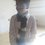# Who's wrong?

So we solved a question in class today:

Find the range of $f(x)=\frac{x}{x^2+1}$.

The general method we're told to follow is to find the inverse of the function and then find the domain of the inverse, which is the range of the function. So this was the solution put up.

Domain of $f$ will be $\mathbb{R}$. Let

\begin{aligned} y&=&\frac{x}{1+x^2} \\ yx^2-x+y &=& 0 \\ x &=& \frac{1\pm\sqrt{1-4y^2}}{2y}......................(1)\end{aligned}

$\therefore I.~1-4y^2\geq0 \Rightarrow y^2\leq\frac{1}{4} \Rightarrow y\in\left[-\frac{1}{2},\frac{1}{2}\right]$

$II.~2y\neq0\Rightarrow y\neq0$

$\therefore y\in\left[-\frac{1}{2},\frac{1}{2}\right]-\{0\}$

Hence the range of $f(x)$ is $\left[-\frac{1}{2},\frac{1}{2}\right]-\{0\}$.

I argued that $f(x)=0$ for $x=0$, and hence $0$ should be a part of the range. But then my teacher told me that $0$ won't be a part of the range, as $y=0$ is not allowed in equation $(1)$. But then I showed the teacher the definition of the range given in my textbook (NCERT), and it was the definition for the range of a relation. He said that the definition of a range is different for relations and different for functions.

Is he wrong or right? If he's wrong, how do I prove him wrong, and if he's right, what did I do wrong?

Wikipedia defines the range of a function as the image of the function, which makes me think that I'm right. Also, the graph of $y=\frac{x}{x^2+1}$ passes through the origin.Note by Omkar Kulkarni
5 years, 9 months ago

This discussion board is a place to discuss our Daily Challenges and the math and science related to those challenges. Explanations are more than just a solution — they should explain the steps and thinking strategies that you used to obtain the solution. Comments should further the discussion of math and science.

When posting on Brilliant:

• Use the emojis to react to an explanation, whether you're congratulating a job well done , or just really confused .
• Ask specific questions about the challenge or the steps in somebody's explanation. Well-posed questions can add a lot to the discussion, but posting "I don't understand!" doesn't help anyone.
• Try to contribute something new to the discussion, whether it is an extension, generalization or other idea related to the challenge.

MarkdownAppears as
*italics* or _italics_ italics
**bold** or __bold__ bold
- bulleted- list
• bulleted
• list
1. numbered2. list
1. numbered
2. list
Note: you must add a full line of space before and after lists for them to show up correctly
paragraph 1paragraph 2

paragraph 1

paragraph 2

[example link](https://brilliant.org)example link
> This is a quote
This is a quote
    # I indented these lines
# 4 spaces, and now they show
# up as a code block.

print "hello world"
# I indented these lines
# 4 spaces, and now they show
# up as a code block.

print "hello world"
MathAppears as
Remember to wrap math in $$ ... $$ or $ ... $ to ensure proper formatting.
2 \times 3 $2 \times 3$
2^{34} $2^{34}$
a_{i-1} $a_{i-1}$
\frac{2}{3} $\frac{2}{3}$
\sqrt{2} $\sqrt{2}$
\sum_{i=1}^3 $\sum_{i=1}^3$
\sin \theta $\sin \theta$
\boxed{123} $\boxed{123}$

Sort by:

A better way to solve this question is find the derivative of the function and equate it to zero. We would get the critical points which are +1/2 and -1/2. Thus zero would be included in the range of f(x). Using the above method is bit more risky as above one may gain extraneous roots or lose important roots.

- 5 years, 9 months ago

True, but again, school. Can't mix up topics, especially ones that we haven't been taught.

- 5 years, 9 months ago

As far as my views are concerned, you are correct. for y=0, equation yields 0/0 which is indeterminate form. But if you look at your quadratic equation, you will find that on putting y=0, equation becomes linear and yields a solution x=0, which is applicable.

Now, by means of this note you have raised a good question, whether y=0 is applicable here or not. A similar type question appeared in JEE Mains this year on which a lot of debate has been on brilliant. I am explaining it with a example.

How many solutions of equation $x^{2}$ = x are there. Obviously, there are two solutions 0 and 1. But how many solutions of equation $x^{2}$/x =1 are there. There is only one solution x=1. Now what's difference between the both, only transfer of x has been from RHS to LHS. But it has changed the domain of original question. In first case, x=0 is allowed in domain, but in second case x=0 is not allowed. Except this condition, both questions are same.

Remember that, every time you want to exact solutions of a problem, work on original question not on any modified part.You are putting y=0 on a modified part of the original question. Put y=0 in quadratic equation and then solve the problem Quadratic formula works for all quadratic equation. But here putting y=0, equation becomes linear not quadratic.

Hope you understand. Ask if you have any other problem.

- 5 years, 9 months ago

Exactly what I think. Thank you! But how do I prove him wrong?

- 5 years, 9 months ago

4th paragraph. You can give example above to support your argument

- 5 years, 9 months ago

Okay thanks!

- 5 years, 9 months ago

$0$ does lie in the range of the function. Why does he say $y \neq 0$ ?

- 5 years, 9 months ago

Exactly! He said that $y\neq0$ as it does not satisfy the inverse.

- 5 years, 9 months ago